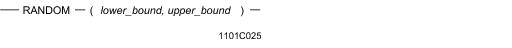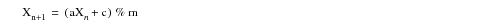# 15.00 - RANDOM - Teradata Database

## Teradata Database SQL Functions, Operators, Expressions, and Predicates

Product
Release Number
15.00
Content Type
Programming Reference
Publication ID
B035-1145-015K
Language
English (United States)

## RANDOM

### Purpose

Returns a random integer number for each row of the results table.

### Syntaxwhere:

 Syntax element … Specifies … lower_bound an integer literal to define the lower bound on the closed interval over which a random number is to be selected. The limits for lower_bound range from -2147483648 to 2147483647, inclusive. lower_bound must be less than or equal to upper_bound. upper_bound an integer literal to define the upper bound on the closed interval over which a random number is to be selected. The limits for upper_bound range from -2147483648 to 2147483647, inclusive. upper_bound must be greater than or equal to lower_bound.

### ANSI Compliance

This is a Teradata extension to the ANSI SQL:2011 standard.

### Result Type and Attributes

The data type, format, and title for RANDOM(x,y) are as follows.

 Data Type Format Title INTEGER Default format for INTEGER Random(x,y)

For information on default data type formats, see SQL Data Types and Literals.

### Computation

RANDOM uses the linear congruential algorithm and 48-bit integer arithmetic.

The algorithm works by generating a sequence of 48-bit integer values, Xi, using the following equation:where:

 This variable … Represents … X a random number over a defined closed interval n an integer >= 0 a 0x5DEECE66D c 0xB % the modulo operator m 248

### Multiple RANDOM Calls Within a SELECT List

You can call RANDOM any number of times in the SELECT list, for example:

`   SELECT RANDOM(1,100), RANDOM(1,100);`

Each call defines a new random value.

### Restrictions

The following rules and restrictions apply to the use of the RANDOM function.

• RANDOM can only be called in one of the following SELECT query clauses:
• WHERE
• GROUP BY
• ORDER BY
• HAVING/QUALIFY
• RANDOM cannot be referenced by position in a GROUP BY or ORDER BY clause.
• RANDOM cannot be nested inside aggregate or ordered analytical functions.
• RANDOM cannot be used in the expression list of an INSERT statement to create a primary index or partitioning column value.
• For example:

`   INSERT t1 (RANDOM(1,10),...) `
` `

RANDOM causes an error to be reported in this case if the first column in the table is a primary index or partitioning column.

### Using RANDOM as a Condition on an Index

Because the RANDOM function is evaluated for each selected row, a condition on an index column that includes the RANDOM function results in an all-AMP operation.

For example, consider the following table definition:

`   CREATE TABLE t1`
`      (c1 INTEGER`
`      ,c2 VARCHAR(9))`
`   PRIMARY INDEX ( c1 );`

The following SELECT statement results in an all-AMP operation:

`   SELECT * `
`   FROM t1 `
`   WHERE c1 = RANDOM(1,12);`

### Example

Suppose you have a table named sales_table with the following subset of columns.

 Store_ID Product_ID Sales 1003 C 20000 1002 C 35000 1001 C 60000 1002 D 50000 1003 D 50000 1001 D 35000 1001 A 100000 1002 A 40000 1001 E 30000

The following SELECT statement returns a random number between 1 and 3, inclusive, for each row in the results table.

`   SELECT store_id, product_id, sales, RANDOM(1,3)`
`   FROM sales_table;`

The results table might look like this.

 Store_ID Product_ID Sales RANDOM(1,3) 1003 C 20000 1 1002 C 35000 2 1001 C 60000 2 1002 D 50000 3 1003 D 50000 2 1001 D 35000 3 1001 A 100000 2 1002 A 40000 1 1001 E 30000 2